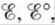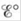Chapter 18, Problem 5RQ

Chapter
Section
Textbook Problem

# The Nernst equation allows determination of the cell potential for a galvanic cell at nonstandard conditions. Write out the Nernst equation. What are nonstandard conditions? What do, n, and Q stand for in the Nernst equation? What does the Nernst equation reduce to when a redox reaction is at equilibrium? What are the signs of ΔG° andwhen K < 1? When K > 1? When K = 1? Explain the following statement:determines spontaneity, whiledetermines the equilibrium position. Under what conditions can you useto predict spontaneity?

Interpretation Introduction

Interpretation:

The significance of the Nernst equation is given. The terms associated with the Nernst equation and the effect of equilibrium over the Nernst equation is to be stated.

Concept introduction:

When the non-standard conditions are given, at that point the Nernst equation is used to determine the relationship between the reduction potential of an electrochemical cell reaction to that of the electrode potential at the standard conditions, given temperature and the activities of the species that are involved in the cell reaction.

Using the E , the spontaneity of the cell reaction can be determined

At equilibrium, the value of E becomes zero, which reduces the Nernst equation to a new form.

To determine: The formula for the Nernst equation; the non-standard conditions for the Nernst equation; the significance of the terms E , E° , n and Q in the Nernst equation; the Nernst equation when the redox reaction approaches equilibrium state; the signs of ΔG° and E° when K<1 , K>1 and K=1 ; the explanation of the statement, “ E determines spontaneity, while E° determines the equilibrium position”.

Explanation

The Nernst equation is defined as,

E = E°-RTnFlnQ

The Nernst equation is used to determine the relationship between the reduction potential of an electrochemical cell reaction to that of the electrode potential at the standard conditions, given temperature and the activities of the species that are involved in the cell reaction.

The significance of the terms used in the equation E=E°RTnFlnQ is as follows,

• E is the potential of the cell at the given temperature.
• E is the standard potential of the cell.
• R is the universal gas constant, (8.314JK1mol1) .
• T is the given temperature in Kelvins .
• Q is the reaction quotient for the cell reaction.
• n is the number of electrons involved in the cell reaction.
• F is the Faraday’s constant.

At the standard conditions , the Nernst equation reduces to,

E = E°-0.0591nlnQ

The conditions other than the standard conditions like 1mol/L concentration and 1atm pressure at the given temperature are the non-standard conditions.

The Nernst equation is used to determine the potential of the cell under the non-standard conditions.

Every term used in Nernst equation has its own significance that determines the conditions involved in the cell reaction.

The Nernst equation is E=E°RTnFlnQ where,

• E is the potential of the cell at the given temperature.
• E° is the standard potential of the cell.
• Q is the reaction quotient for the cell reaction.
• n is the number of electrons involved in the cell reaction.

The Nernst equation at equilibrium state approaches to the equation,

lnK = nFE°RT

At equilibrium E=0 and Q=K

Substitute these values in the Nernst equation as,

0 = E°-RTnFlnKlnK = nFE°RT

The above equation is used to determine the equilibrium constant for the given reaction

### Still sussing out bartleby?

Check out a sample textbook solution.

See a sample solution

#### The Solution to Your Study Problems

Bartleby provides explanations to thousands of textbook problems written by our experts, many with advanced degrees!

Get Started